# Absolute reference calculation issue

Hi, my question is  how i can sum all "True" members in calculate column (see attached image) i'm using the follow formula but isn't works:

sum(@"";"[True]"(l(1,0)), 0, m1)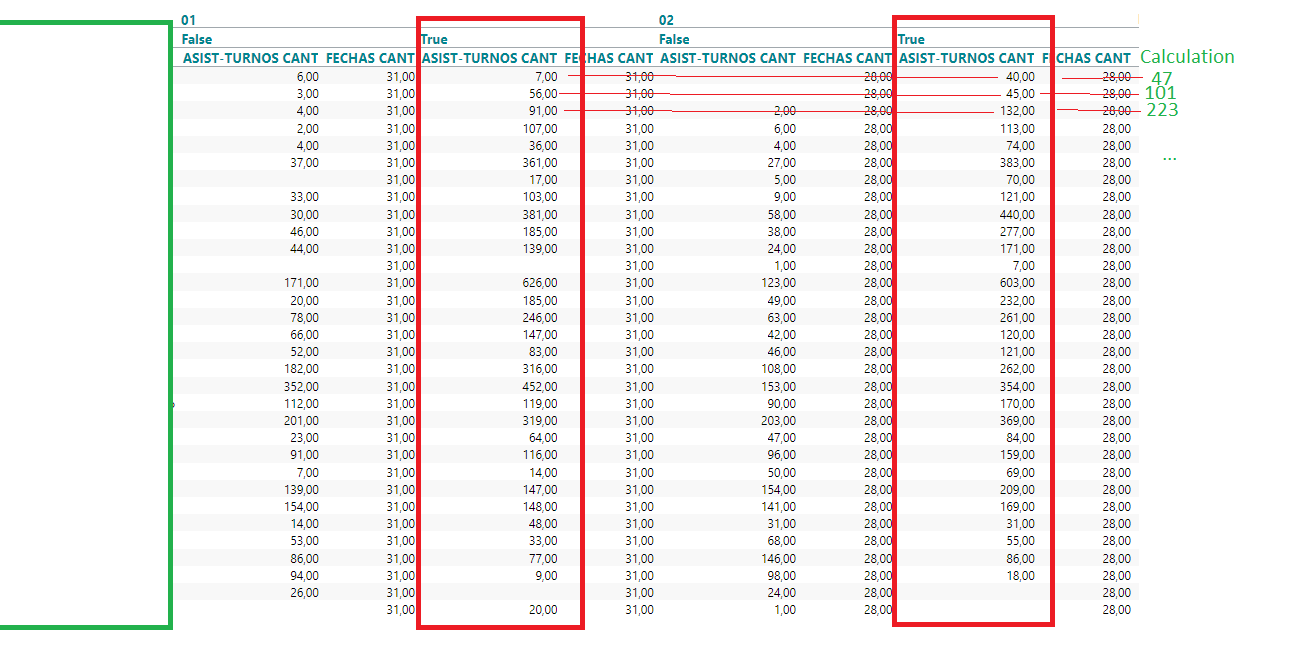• Hi Jean Pierre

I guess you are trying to add a sort of wildcard on the first level - marked in bold below

sum(@"";"[True]"(l(1,0)), 0, m1)

That isn't supported in the syntax - which makes your problem a little complex.

However - I found a solution - based on the assumption that there will always be a "TRUE" and "FALSE" for each top level of your table?

My solution involves one intermediate calculation, which can be hidden once the end result is calculated.

I've created this table from demodata to create something like your issue: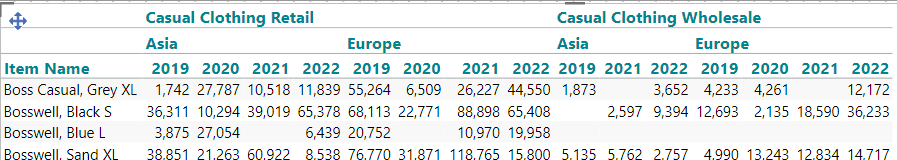In my case I want to add up the totals of Europe (which I think is pretty much the same type of problem you are facing)

My first intermediate calculation could be an allcount of level 2's. In my table - Companies are level 1 - Territories level 2 and Years is level 3.

So I will try an add a new measure with this syntax:

allcount(d1:0(l(1,1,0)), d1, m1)

As you can see in the table below - the count goes 1 up every time we meat a new Territory.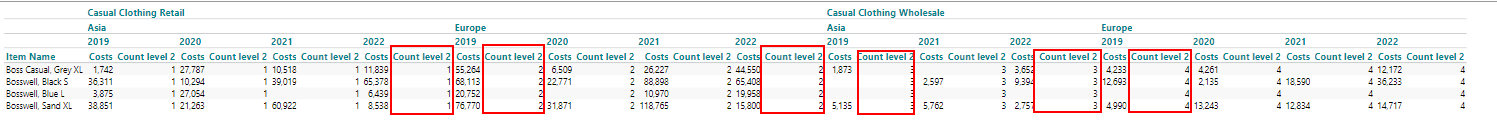Now we can refine this calculation a bit:

mod(allcount(d1:0(l(1,1,0)), d1, m1);2)

The mod function divides the number calculated with allcount by 2 and only keeps the remainder, which gives us this result: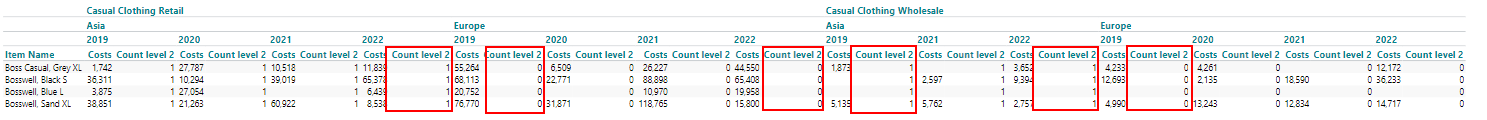The remainder will alternate between 1 and 0 depending on is the allcount has reached an even or an odd number.

Let's refine the calculation even more:

if mod(allcount(d1:0(l(1,1,0)), d1, m1);2) = 0 then sum(0,0,m1) else 0

Now we are making a new measure (m2) which will only contain numbers for Europe: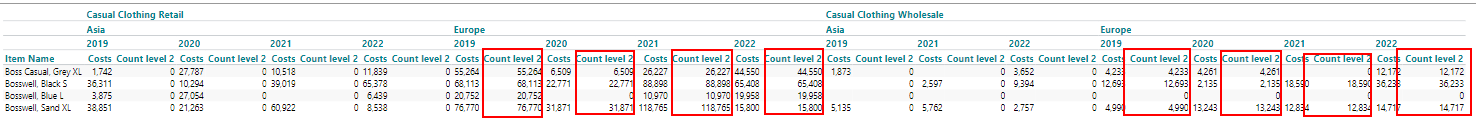Having done this, we just need to add a calcuated column with the syntax:

sum(all,0,m2)

And go to properties, visibility and hide our intermediate calculation.

I hope it makes sense :-)

br/Niels

Btw: If the screenshots are a bit small - just zoom in your browser

• Thank you very much, I was very helpful !